Courses

# Introduction: The Moment Distribution Method - 1 Civil Engineering (CE) Notes | EduRev

## Civil Engineering (CE) : Introduction: The Moment Distribution Method - 1 Civil Engineering (CE) Notes | EduRev

The document Introduction: The Moment Distribution Method - 1 Civil Engineering (CE) Notes | EduRev is a part of the Civil Engineering (CE) Course Structural Analysis.
All you need of Civil Engineering (CE) at this link: Civil Engineering (CE)

Instructional Objectives

After reading this chapter the student will be able to

1. Calculate stiffness factors and distribution factors for various members in a continuous beam.
2. Define unbalanced moment at a rigid joint.
3. Compute distribution moment and carry-over moment.
4. Derive expressions for distribution moment, carry-over moments.
5. Analyse continuous beam by the moment-distribution method

Introduction

In the previous lesson we discussed the slope-deflection method. In slopedeflection analysis, the unknown displacements (rotations and translations) are related to the applied loading on the structure. The slope-deflection method results in a set of simultaneous equations of unknown displacements. The number of simultaneous equations will be equal to the number of unknowns to be evaluated. Thus one needs to solve these simultaneous equations to obtain displacements and beam end moments. Today, simultaneous equations could be solved very easily using a computer. Before the advent of electronic computing, this really posed a problem as the number of equations in the case of multistory building is quite large. The moment-distribution method proposed by Hardy Cross in 1932, actually solves these equations by the method of successive approximations. In this method, the results may be obtained to any desired degree of accuracy. Until recently, the moment-distribution method was very popular among engineers. It is very simple and is being used even today for preliminary analysis of small structures. It is still being taught in the classroom for the simplicity and physical insight it gives to the analyst even though stiffness method is being used more and more. Had the computers not emerged on the scene, the moment-distribution method could have turned out to be a very popular method. In this lesson, first moment-distribution method is developed for continuous beams with unyielding supports.

Basic Concepts

In moment-distribution method, counterclockwise beam end moments are taken as positive. The counterclockwise beam end moments produce clockwise moments on the joint Consider a continuous beam ABCD as shown in Fig.18.1a. In this beam, ends A and D are fixed and hence,θA = θD = 0 .Thus, the deformation of this beam is completely defined by rotations θB and θC at joints B and C respectively. The required equation to evaluate θB and θC is obtained by considering equilibrium of joints B and C. Hence,

∑ MB = 0 ⇒ MBA + MBC = 0                                       (18.1a)

∑ MC = 0 ⇒ MCB + MCD = 0                                      (18.1b)

According to slope-deflection equation, the beam end moments are written as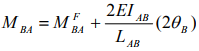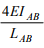is known as stiffness factor for the beam AB and it is denoted by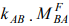is the fixed end moment at joint B of beam AB when joint B is fixed.

Thus,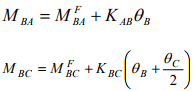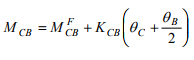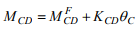(18.2)

In Fig.18.1b, the counterclockwise beam-end moments MBA and MBC produce a clockwise moment MB on the joint as shown in Fig.18.1b. To start with, in moment-distribution method, it is assumed that joints are locked i.e. joints are prevented from rotating. In such a case (vide Fig.18.1b),

θB = θC = 0, and hence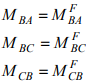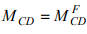(18.3)

Since joints B and C are artificially held locked, the resultant moment at joints B and C will not be equal to zero. This moment is denoted MB by and is known as the unbalanced moment.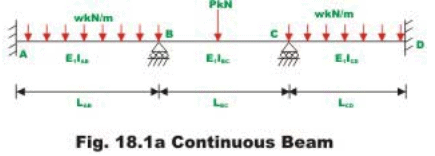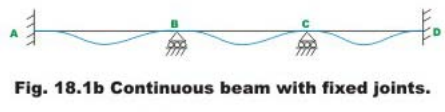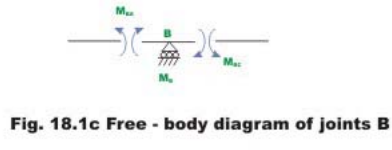Thus,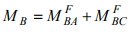In reality joints are not locked. Joints B and C do rotate under external loads. When the joint B is unlocked, it will rotate under the action of unbalanced moment MB. Let the joint B rotate by an angle θB1 , under the action of MB. This will deform the structure as shown in Fig.18.1d and introduces distributed moment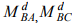in the span BA and BC respectively as shown in the figure. The unknown distributed moments are assumed to be positive and hence act in counterclockwise direction. The unbalanced moment is the algebraic sum of the fixed end moments and act on the joint in the clockwise direction. The unbalanced moment restores the equilibrium of the joint B. Thus,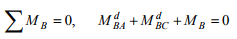(18.4)

The distributed moments are related to the rotation θB1 by the slope-deflection equation.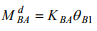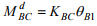(18.5)

Substituting equation (18.5) in (18.4), yields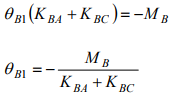In general,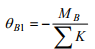(18.6)

where summation is taken over all the members meeting at that particular joint. Substituting the value of θB1 in equation (18.5), distributed moments are calculated. Thus,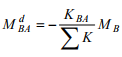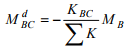(18.7)

The ratio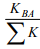is known as the distribution factor and is represented by DFBA.

Thus,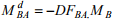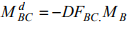(18.8)

The distribution moments developed in a member meeting at B, when the joint B is unlocked and allowed to rotate under the action of unbalanced moment MB is equal to a distribution factor times the unbalanced moment with its sign reversed.

As the joint B rotates under the action of the unbalanced moment, beam end moments are developed at ends of members meeting at that joint and are known as distributed moments. As the joint B rotates, it bends the beam and beam end moments at the far ends (i.e. at A and C) are developed. They are known as carry over moments. Now consider the beam BC of continuous beam ABCD.

When the joint B is unlocked, joint C is locked .The joint B rotates by θB1 under the action of unbalanced moment MB (vide Fig. 18.1e). Now from slopedeflection equations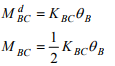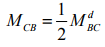(18.9)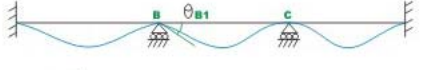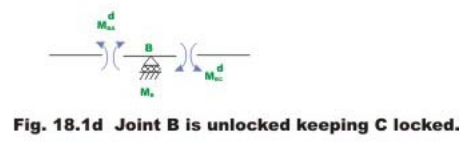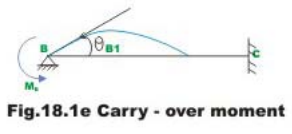The carry over moment is one half of the distributed moment and has the same sign. With the above discussion, we are in a position to apply momentdistribution method to statically indeterminate beam. Few problems are solved here to illustrate the procedure. Carefully go through the first problem, wherein the moment-distribution method is explained in detail.

Offer running on EduRev: Apply code STAYHOME200 to get INR 200 off on our premium plan EduRev Infinity!

## Structural Analysis

30 videos|122 docs|28 tests

,

,

,

,

,

,

,

,

,

,

,

,

,

,

,

,

,

,

,

,

,

;